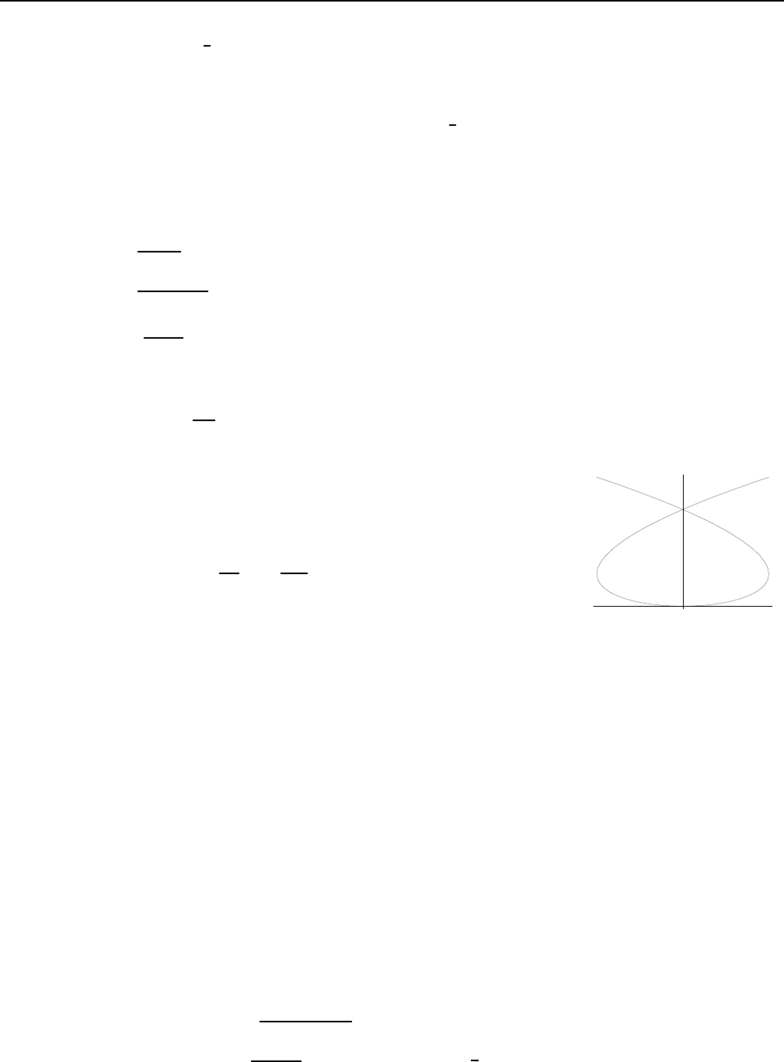# MATH 222 Study Guide - Maxima And Minima, Nappe, Cylindrical Coordinate System

18 views4 pages
School
McGill University
Department
Mathematics & Statistics (Sci)
Course
MATH 222
Page:
of 4201-DDB Final Exam — May 2011
(Marks)
Calculus III Page 1
1. Let f(x) = Zx
0
tcos t dt:(6)
(a) ﬁnd a power series representation for f(x);
(b) use this series to approximate f(x) = Z1/2
0
tcos t dt correctly to 4 decimal places.
2. Find the power series representation for each of the following functions, and state the radius of(6)
convergence.
(a) f(x) = 1
43x, centered at x= 2.
(b) f(x) = 3
2 + xx2, centered at x= 0.
3. Let f(x) = 3
8 + x:(8)
(a) use the Binomial theorem to ﬁnd the ﬁrst 5 terms of the Maclaurin series for f(x), and its radius
of convergence;
(b) approximate 3
8.2 correctly to 4 decimal places.
4. Let Cbe the plane curve deﬁned by parametric equations (x= 3tt3
y= 3t2
(8)
(a) Show the orientation of C.
(b) Find and simplify dy
dx and d2y
dx2.
(c) At what points does Chave a vertical tangent line?
(d) Set up (but do not evaluate) the integral needed to ﬁnd the area
of the region enclosed by the loop.
5. (a) Sketch the graph of r= 2 sin(3θ).(6)
(b) Find the area of the region enclosed by the curve.
(c) Set up (but do not evaluate) the integral needed to ﬁnd the length of one loop of the curve.
6. Let Cbe the space curve deﬁned by the vector equation r(t) = het,etsin t, etcos ti.(10)
(a) Find the equation of a quadric surface on which Clies. Sketch both the surface and the curve.
(b) Find the unit tangent vector Tand the unit normal vector N.
(c) Find the length of Con the interval 0 t1.
(d) Find the curvature κof C.
(e) Find the parametric equations of the tangent line to Cat the point where t= 0.
7. Sketch and describe the following. Show all your work.(9)
(a) The surface f(x, y) = px2+ 2y2+ 1.
(b) The level curve of z=y
x2+y2corresponding to z=1
4.
(c) The surface ρ= csc ϕcot ϕ.
201-DDB Final Exam — May 2011
(Marks)
Calculus III Page 2
8. Let rbe a three-times-diﬀerentiable function of t. Simplify: [r·(r×r)].(2)
9. Find the limit (or if appropriate, show that it does not exist):(4)
(a) lim
(x,y)(0,0)
x
px2+y2(b) lim
(x,y)(0,0)(x2+y2) ln(x2+y2)
10. Show that if f(t) is diﬀerentiable, then z=f(x/y) is a solution of the partial diﬀerentiable equation(3)
xz
x +yz
y = 0.
11. Let Cbe the curve formed by the intersection of the level surface x2y+yz +z2+ 1 = 0 and the plane(3)
x+y+z= 1. Let P0(1,1,1) be a point on C. Find a tangent vector to Cat P0.
12. Let z=f(x, y) be implicitly deﬁned by sin(xy) + xz4+y3z= 2, and let P0(0,1,2) be a point on this(6)
surface.
(a) Find the equation of the tangent plane to the surface at P0.
(b) Find f(0,1).
(c) Find an approximation of f(0.05,1.10).
13. Find and classify the critical points of f(x, y) = y2+x2y+x22y.(5)
14. Use Lagrange Multipliers to ﬁnd the points on the sphere x2+y2+z2= 3 where the maximum and(5)
minimum values of the product xyz are found.
15. Evaluate:(8)
(a) Z1
1Z1y2
1y2ln(x2+y2+ 1) dx dy (b) Z4
0Z1
0Z2
2y
cos(x2)
zdx dy dz
16. Sketch the solid region Sbounded below by z=px2+y2, and bounded above by ρ= 2 cos φ.(5)
Find the volume of S.
17. Sketch the solid region Sbounded below by the plane z= 0, laterally by the surface x2+(y1)2= 1,(6)
and above by the surface z=x2+y2.
Set up the triple integrals representing the volume of Sin
(a) cartesian coordinates (b) cylindrical coordinates
201-DDB Final Exam–May 2011 ANSWERS Page 1 of 2
1. (a) f(x) =
X
n=0
(1)nxn+2
(n+ 2)(2n)!
(b) f(1
2)1
81
48 +1
1536 0.1048
2. (a) f(x) =
X
n=0
(1)n+13n(x2)n
2n+1 , and R=2
3
(b) f(x) =
X
n=0 1
2n+1 + (1)nxn, and R= 1
3. (a) f(x) = 2 + x
12 x2
288 +5x3
20736 5x4
248832 +···and R= 8
(b) f(0.2) = 2 + 0.2
12 (0.2)2
288 2.0165
with absolute value of error less than 5(0.2)3
20736 = 0.19 ×105
4. (a) Counterclockwise orientation
(b) dy
dx =2t
1t2and d2y
dx2=2(1 + t2)
3(1 t2)3
(c) Vertical tangents at (±2,3)
(d) A= 2 Z3
0
xdy = 2 Z3
0
(3tt3)(6t)dt = 12 Z3
0
(3t2t4)dt
5. (a)
x
y
(b) A=Zπ
0
1
2(2 sin(3θ))2=π
(c) L=Zπ/3
0p4 + 32 cos2(3θ)= 2 Zπ/3
0p1 + 8 cos2(3θ)
6. (a) The curve lies on the cone x2=y2+z2. Note that x=etso x > 0 implying that the curve
spirals around x=py2+z2, the upper nappe of the cone.
(b) T(t) = 1
3h1,sin t+ cos t, cos tsin ti
and N(t) = 1
2h0,cos tsin t, sin tcos ti
(c) L=3(e1)

## Document Summary

Page 1 (marks) (6: let f (x) = z x. 0 t cos t dt: (a) nd a power series representation for f (x); (b) use this series to approximate f (x) = z 1/2. 0 t cos t dt correctly to 4 decimal places. (6: find the power series representation for each of the following functions, and state the radius of convergence. (a) f (x) = (b) f (x) = Show all your work. (a) the surface f (x, y) = px2 + 2y2 + 1. (b) the level curve of z = y x2 + y2 corresponding to z = 1. 4 . (c) the surface = csc cot . = 0. (3: let c be the curve formed by the intersection of the level surface x2y + yz + z2 + 1 = 0 and the plane x + y + z = 1. 1z ln(x2 + y2 + 1) dx dy (b) z 4.